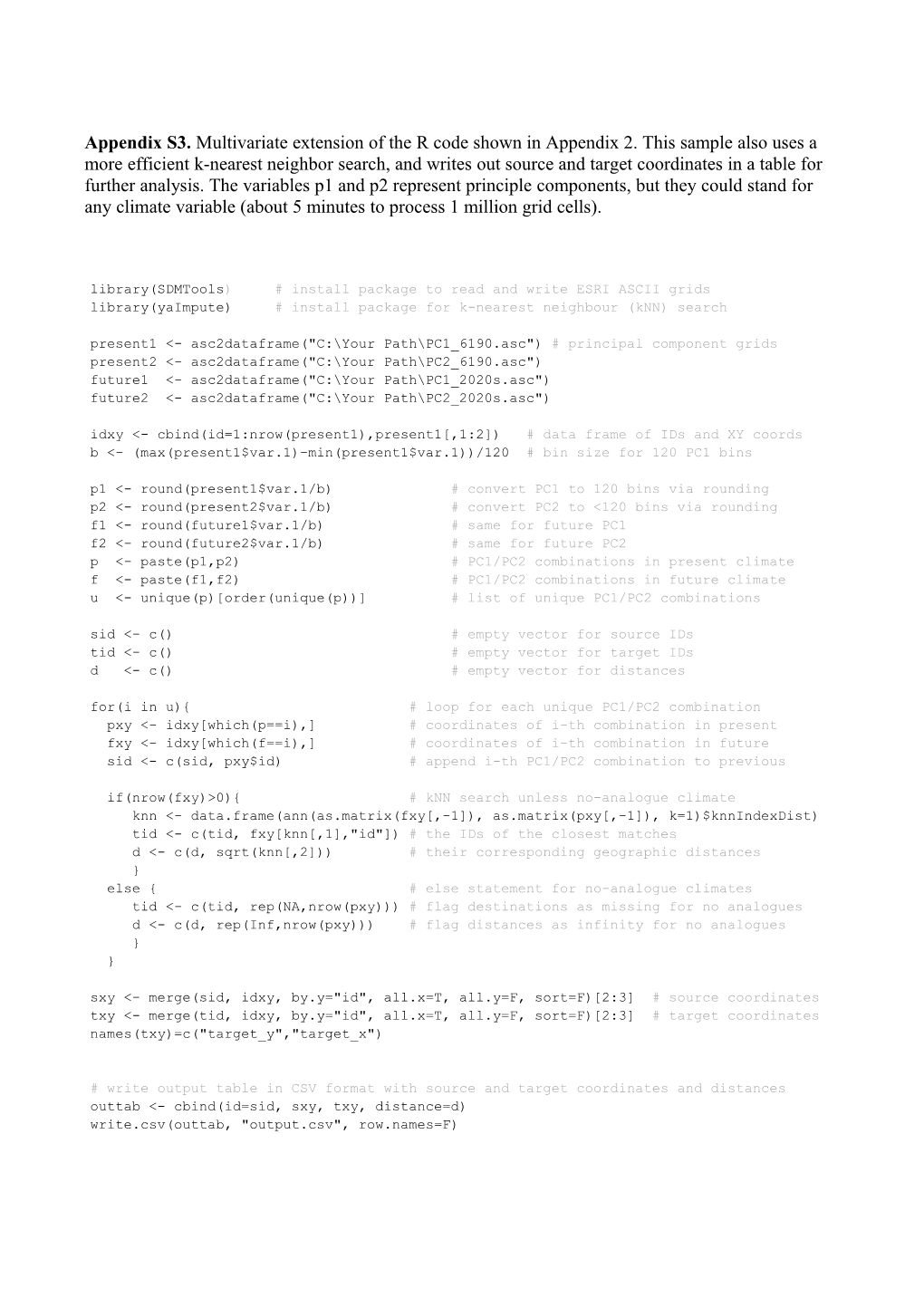# Library(Sdmtools) # Install Package to Read and Write ESRI ASCII GridsAppendix S3.Multivariate extension of the R code shown in Appendix 2. This sample also uses a more efficient k-nearest neighbor search, and writes out source and target coordinates in a table for further analysis.The variables p1 and p2 represent principle components, but they could stand for any climate variable (about 5 minutes to process 1 million grid cells).

library(SDMTools) # install package to read and write ESRI ASCII grids

library(yaImpute) # install package for k-nearest neighbour (kNN) search

present1 <- asc2dataframe("C:\Your Path\PC1_6190.asc") # principal component grids

idxy <- cbind(id=1:nrow(present1),present1[,1:2]) # data frame of IDs and XY coords

b <- (max(present1\$var.1)-min(present1\$var.1))/120 # bin size for 120 PC1 bins

p1 <- round(present1\$var.1/b) # convert PC1 to 120 bins via rounding

p2 <- round(present2\$var.1/b) # convert PC2 to <120 bins via rounding

f1 <- round(future1\$var.1/b) # same for future PC1

f2 <- round(future2\$var.1/b) # same for future PC2

p <- paste(p1,p2) # PC1/PC2 combinations in present climate

f <- paste(f1,f2) # PC1/PC2 combinations in future climate

u <- unique(p)[order(unique(p))] # list of unique PC1/PC2 combinations

sid <- c() # empty vector for source IDs

tid <- c() # empty vector for target IDs

d <- c() # empty vector for distances

for(i in u){ # loop for each unique PC1/PC2 combination

pxy <- idxy[which(p==i),] # coordinates of i-th combination in present

fxy <- idxy[which(f==i),] # coordinates of i-th combination in future

sid <- c(sid, pxy\$id) # append i-th PC1/PC2 combination to previous

if(nrow(fxy)>0){ # kNN search unless no-analogue climate

knn <- data.frame(ann(as.matrix(fxy[,-1]), as.matrix(pxy[,-1]), k=1)\$knnIndexDist)

tid <- c(tid, fxy[knn[,1],"id"]) # the IDs of the closest matches

d <- c(d, sqrt(knn[,2])) # theircorrespondinggeographic distances

}

else { # else statement for no-analogue climates

tid <- c(tid, rep(NA,nrow(pxy))) # flag destinations as missing for no analogues

d <- c(d, rep(Inf,nrow(pxy))) # flag distances as infinity for no analogues

}

}

sxy <- merge(sid, idxy, by.y="id", all.x=T, all.y=F, sort=F)[2:3] # source coordinates

txy <- merge(tid, idxy, by.y="id", all.x=T, all.y=F, sort=F)[2:3] # target coordinates

names(txy)=c("target_y","target_x")

# write output table in CSV format with source and target coordinates and distances

outtab <- cbind(id=sid, sxy, txy, distance=d)

write.csv(outtab, "output.csv", row.names=F)

# writes out log10 velocities and distances multiplied by 100 in ESRI ASCII format

# conversion: -200=0.01km, -100=0.1km, 0=1km, 100=10km, 200=100km etc.

out=merge(present1[,1:2], outtab[,c(2,3,6)], by=c("y","x"), sort=F)

out\$distance[out\$distance==Inf] <- 10000 # sets no analogue to 10,000km

out\$distance[out\$distance==0] <- 0.5 # sets zero distance to 0.5km (1/2 cell size)

out\$logDist=round(log10(out\$distance)*100)

out\$logSpeed=round(log10(out\$distance/50)*100)

dataframe2asc(out)